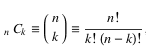# Project Euler – Problem 15 Solution

Presently sponsored by Serverless Guru: Your guide to cloud excellence, helping you every step of your serverless journey, including team training, pattern development, mass service migrations, architecting, and developing new solutions. Speak to a Guru today.

#### Problem

Starting in the top left corner of a 2×2 grid, there are 6 routes (without backtracking) to the bottom right corner.How many routes are there through a 20×20 grid?

#### Solution

```let rec factorial(n:bigint) = if n <= 1I then 1I else n * factorial(n-1I)
let combo n k = factorial(n) / (factorial(k) * factorial(n-k))
let answer = combo 40I 20I
```

It took me a while to figure out that this problem is actually a simple combination problem – consider a X by Y grid, any route from the top left to the bottom right corner without backtracking must have travelled Right X number of times and Down Y number of times. In the case of the original example:

RRDD, RDRD, RDDR, DRRD, DRDR, DDRR

This also means that all routes have a total of X + Y steps, and the number of routes is equal to the number of ways you can pick X number of R moves out of X + Y, i.e.where n = X + Y and k = X.

### 1 thought on “Project Euler – Problem 15 Solution”

1.let p15 =
let factorial n = [1I..n] |> List.fold (*) 1I
factorial(2I*20I)/(factorial(20I) ** 2)